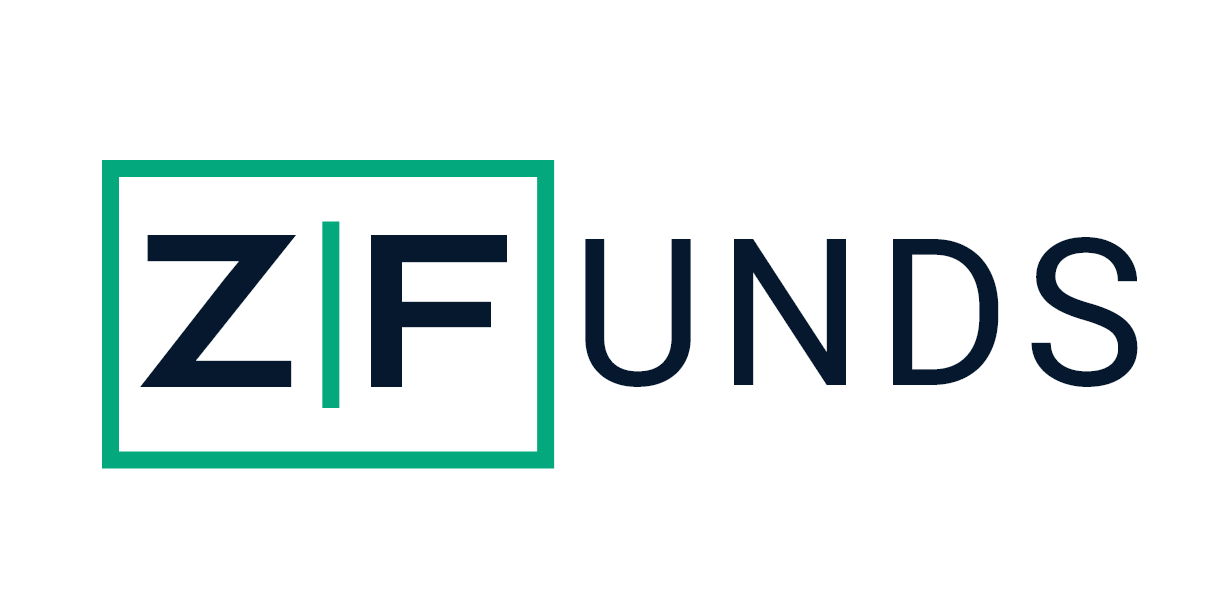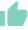# Yield to Maturity (YTM): Meaning, Maturity, Formula, Importance and LimitationsManish Kothari
20 Likes | 845 days agoLikeShareAuthor is a Zfunds Verified ExpertManish Kothari
Gurugram## WHAT IS YTM?

Yield to Maturity (YTM) is the overall return anticipated on a bond if the bond is held until its maturity. YTM is considered as a long-run bond yield but is conveyed as an annual rate. In simpler words, it is the IRR (Internal Rate of Return) of an investment in a bond if the bondholder holds the bond till its expiry or maturity, with all payments made as scheduled and reinvested at the same return rate. "Redemption yield" or "Book yield" are other names of YTM.

## Important Terms in YTM

1. Par Value/ Face Value:

Par value or Face value is the value of the bond upon expiry. In simpler words, this is the price paid to the investor by the bond issuer at the maturity date.

2. Market Value/ Present Value:

The market Value or Present value of the bond is the current market price. The bond prices are subject to variation on the basis of the interest rate changes in the economy. Both yield and price of bonds have an inverse relationship.

3. Coupon Rate:

The coupon rate is the interest rate paid to the investor by the bond issuer. The interest rate is paid on the bond’s par/face value and not on the present/market value.

4. Interest Rate:

The interest rate of a bond is not the same as its coupon rate. Let’s understand this with an illustration. Mr. Lakshay purchases a bond at Rs. 10,000 (face value), and the coupon rate is 10%. Mr. Lakshay gets Rs. 1,000 (10% of Rs. 10,000) per annum for his investment as annual coupon payments. The effective interest rate for lakshay is 10%.

On the other side, Ms. Swati buys a bond at Rs. 20,000 (at a higher price to its par/face value). The face value of the bond is Rs 10,000, and the coupon rate is 10%. Here, Ms. Swati also gets an annual payment of Rs. 1,000 (10% of Rs. 10,000) as yearly coupon payments. But, since she bought the bond at Rs. 20,000, the rate of interest for her investment is 5% (Rs. 1000 of Rs. 20,000).

Identically, had an individual bought the bond below its par/face value, the interest rate would be more than that of the coupon rate.

Face value < Market value, it means that the bond is trading at a premium

Market value = Face value, it means that the bond is trading at par

Face value > Market value, it means that the bond is trading at a discount

6. Time to Maturity:

Maturity is the tenure, period, or date when a bond’s principal amount is repaid along with the interest. For instance, a 15-year government bond matures in 15 years. The investor receives the principal amount clubbed with interest at that time. Often, maturity is called the time to maturity. This conveys the period of time between the present and the bond maturity date.

## HOW YTM IS CALCULATED?

YTM is typically expressed as an APR i.e. Annual Percentage Rate. It is calculated through the use of the following formula:

YTM = [C+(FV-PV)/T]/[(FV+PV)/2]

Where:

• C – Coupon/Interest payment
• FV – Face Value of the bond
• PV – Price/Present Value of the bond
• T – How much time (years) it takes the bond to reach its maturity

The formula’s aim is to determine the yield of a bond (or any other fixed-asset security) according to its most recent market/current price. The YTM calculation is structured to show the effective yield of the security once it reaches maturity (based on compounding).

## How does YTM Works?

To understand YTM in a better way and how it works, let’s look at an illustration.

Assume that there is a bond listed on the market priced at Rs. 850 and that the bond comes with a Par/Face value of Rs. 1,000. On this particular bond, yearly coupons are Rs. 150. The coupon rate for the bond is 15% and the bond will reach maturity in seven years.

The formula to calculate YTM would be:

YTM = [150+(1000-850)/7]/[(1000+850)/2]

The YTM on the bond comes out to be 18.53%.

## IMPORTANCE OF YTM

YTM assists in deciding whether buying bonds is an ideal investment option or not. Also, YTM is a popular metric used for drawing comparisons between bonds & securities. In simpler words, YTM assists investors to compare returns from various securities. It helps in the selection and shortlisting of the securities one can invest in as per their expected returns.

YTM is expressed at a yearly/annual rate. Thus, irrespective of the maturity of the bond, YTM can be used to compare bonds with varying coupons and maturities.

Adding to it, YTM also helps in understanding the change in prices of the securities with respect to the changing market scenarios like movement in interest rates & others. The yields and bond price share an inverse relationship. For instance, with a fall in yield of the securities, the bond prices rise, and vice versa.

## LIMITATIONS OF YTM

• YTM doesn’t take taxes into consideration that investors would have to pay on their investments.
• YTM calculations do not take the costs associated with the purchase and selling of the bonds into consideration.
• The YTM calculations are based on certain assumptions about the future. Estimated YTM doesn’t account for the following:
1. The bond issuing body might default on payments.
2. The bondholder might not be able to reinvest all the coupon payments.
3. One may not be able to hold the securities until maturity.

## THE CRUX

YTM can be used as a metric to decide whether to invest in a bond or not. It can assist in deciding if a bond is worth investing in or not on the basis of its expected returns. Investors can compare the YTMs of different bonds to analyze which one has a better road to maturity. The significant point to know is that YTM just indicates expected returns. Even though bonds are far less volatile than shares, nothing in the universe of investments can be taken by the stone. The bond issuer might default on their repayments or interest payments. Another assumption is that the coupon payments are reinvested at a rate equal to YTM. However, there might be the case the investor is not able to obtain that rate or find any investment worth doing. So, make an informed decision and also look at other factors while making investments.